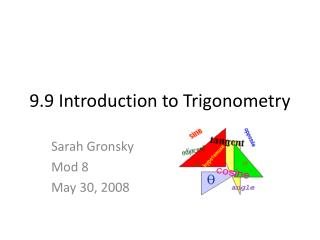# 9.9 Introduction to Trigonometry - PowerPoint PPT PresentationDownload Presentation9.9 Introduction to Trigonometry

9.9 Introduction to TrigonometryDownload Presentation## 9.9 Introduction to Trigonometry

- - - - - - - - - - - - - - - - - - - - - - - - - - - E N D - - - - - - - - - - - - - - - - - - - - - - - - - - -
##### Presentation Transcript

1. 9.9 Introduction to Trigonometry Sarah Gronsky Mod 8 May 30, 2008

2. Introduction • This section will cover the basics of trigonometry. • This section will start off with the sine, cosine, and tangent ratios.

3. Three Trigonometric Ratios B Sine of A = sin of A opposite leg A Hypotenuse C Cosine of A = cos of A Tangent of A = tan of A adjacent leg opposite leg hypotenuse hypotenuse

4. Sample Problems Problem 1. A Find: a. cos A b. tan C 5 13 B C 12

5. Sample Problems Solution 1. A a. cos A = = 5 13 b. tan C = B 12 C =

6. Sample Problems • Problem 2 Find: a. Three trigonometric ratios for < A C b. Three trigonometric ratios for < C 8 17 B 15 A

7. Sample Problem • Problem 2 a. sin < A = C cos < A = tan < A = 8 17 b. sin < C = cos < C = B A tan < C = 15

8. Sample Problems Problem 3 Use the fact that 40˚ 0.6428 to find the height of the moon to the nearest foot. 40˚ 70’

9. Sample Problems • Problem 3 tan 40˚ = 0.6428 44.996 h h 45 ft. 40˚

10. Practice Practice 1. Practice 2. H x J 40˚ 35˚ K N I 3 63˚ J Find x. *If MN is 12 find JK

11. Practice S N U y T V R 40˚ 30˚ A x T E < SVT =40˚ TE = 10 < SRT= 20˚ Find x and y ST = 45 Find UV and RV

12. Answers 1. x 6.6 2. JK 31.4 3. RV 70.0 UV 23.9 4. X 22.1 y 18.5

13. Works Cited • "Math Warehouse." Sine, Cosine and Tangent. Math Warehouse. 22 May 2008 <http://www.mathwarehouse.com/trigonometry/sine-cosine-tangent-practice3.html>. • Rhoad, Richard, George Milauskas and Robert Whipple. Geometry: For Enjoyment and Challenge. New Addition. Evanston, IL: McDougal Little & Compony, 1991.